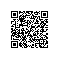# 我得扼住Python的喉咙！等到它对我下手就晚了Python支持布尔类型的数据，布尔类型只有True和False两种值，但是布尔类型有以下几种运算。

1．与运算：只有两个布尔值都为True时，计算结果才为True。

True and True # ==> True
True and False # ==> False
False and True # ==> False
False and False # ==> False
2．或运算：只要有一个布尔值为True，计算结果就是True。

True or True # ==> True
True or False # ==> True
False or True # ==> True
False or False # ==> False
3．非运算：把True变为False，或者把False变为True。

not True # ==> False
not False # ==> True

# 布尔类型

a = True
print(a and 'a=T' or 'a=F')

a=T

‘I am a boy’

"欢迎你加入Python实战圈"

'''

'''

# 转意字符串(n)

command = 'Let's go!'
print('n使用转义字符输出 ：',command)

1．添加空白

# 制表符可以组合使用

print("欢迎来到Python实战圈,n")
print('t你想要学习 Python 的哪方面内容，请留言。')

2．拼接字符串

# 拼接字符串

log_1_str = 'The error is a bug.'
log_2_str = ' We should fix it.'
log_str = log_1_str + log_2_str
print('n拼接后的字符串就是：',log_str)

1．修改字符串的大小写

# 字符串大小写转换

welcome = 'Hello, welcome to Python practical circle'

# title()，每个单词的首字母大写

print('n每个单词的首字母大写：', welcome.title())

# capitalize()，段落的首字母大写

print('n段落的首字母大写：',welcome.capitalize())

# lower()，所有字母小写

print('n所有字母小写：',welcome.lower())

# upper()，所有字母大写

print('n所有字母大写：',welcome.upper())

# 大写转小写，小写转大写

print('n大写转小写，小写转大写：',welcome.swapcase())

# String.isalnum()，判断字符串中是否全部为数字或者英文，符合就返回True，不符合就返回False，如果里面包含符号或者空格之类的特殊字符，那么也会返回False

print('n判断字符串是否全部为数字或者英文：',welcome.isalnum())

# String.isdigit()，判断字符串中是否全部为整数

print('n判断字符串中是否全部为整数：', welcome.isdigit())

2．删除字符串两端的空白

# 删除两端的空白
love_Python = ' Hello, Python Practical Circle '
# 删除字符串两端的空白
print('删除字符串两端的空白',love_Python.strip())
# 删除字符串右侧的空白
print('删除字符串右侧的空白',love_Python.rstrip())
# 删除字符串左侧的空白
print('删除字符串左侧的空白',love_Python.lstrip())

3．其他注意事项

Python中字符串的操作非常多，以上只列出了部分常用操作。有一点需要注意的是，Python中的字符串不允许修改值，只允许覆盖值。也就是说，字符串只能重新赋值。

• [:] 提取从开头（默认位置0）到结尾（默认位置-1）的整个字符串
• [start:] 从start提取到结尾
• [:end] 从开头提取到end-1
• [start:end] 从start 提取到end-1
• [start:end:step] 从start提取到end-1，每step个字符提取一个
• 左侧第一个字符的位置/偏移量为0，右侧最后一个字符的位置/偏移量为-1

# 字符串切片
word = 'Python'
print(word[1:2])
print(word[-2:])
print(word[::2])
print(word[::-1])


y
on
Pto
nohtyP


type()函数在实际项目中经常用到，因为只有知道了变量是什么类型才可以进行相应的运算，比如字典类型和列表类型有不同的运算。类型转换在项目实战中也经常用到，例如一个超市的月销售额是一个字符类型，转换为数字类型才可以进行统计，如计算平均数等，具体的转换语法如下所示。

float(a) 将变量a转换为浮点数
int(b) 将变量b转换为整数
str(c)将变量c转换为字符串

'''

'''
print('n各个数值类型的转换')
number = 100

# number的数据类型是整型，用int表示

print('number的数据类型是：')
print(type(number))

# 将整数转换为浮点数

float_number = float(number)
print('nfloat_number的数据类型是:')
print(type(float_number))

# 将整型转换为字符串

print('nnumber转换为字符串类型')
str_number = str(number)
print('str_number的数据类型是:')
print(type(str_number))

# 将字符串转换为整型int()或者浮点数float()

print('nstr_number转换为数字类型')
int_str_number = int(str_number)
float_str_number = float(str_number)
print('int_str_number的数据类型是：')
print(type(int_str_number))
print('float_str_number的数据类型是:')
print(type(float_str_number))

number的数据类型是：

float_number的数据类型是：

number转换为字符串类型
str_number的数据类型是:

str_number转换为数字类型
int_str_number的数据类型是：

float_str_number的数据类型是:使用钉钉扫一扫加入圈子
+ 订阅